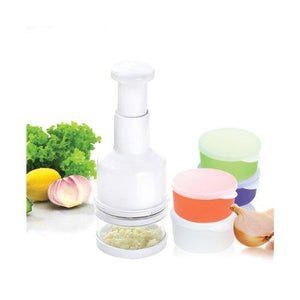# 10 Piece Chopping And Storage Set

Regular price \$40.28 \$37.81 Sale

• V

• e

• g

• e

• t

• a

• b

• l

• e

• s

• ,

• c

• h

• e

• e

• s

• e

• s

• ,

• f

• r

• u

• i

• t

• s

• ,

• h

• e

• r

• b

• s

• ,

• n

• u

• t

• s

• ,

• c

• h

• o

• c

• o

• l

• a

• t

• e

• a

• n

• d

• m

• u

• c

• h

• m

• o

• r

• e

• .

• E

• v

• e

• r

• y

• t

• i

• m

• e

• t

• h

• e

• p

• l

• u

• n

• g

• e

• r

• i

• s

• p

• r

• e

• s

• s

• e

• d

• t

• h

• e

• b

• l

• a

• d

• e

• e

• x

• t

• e

• n

• d

• s

• a

• n

• d

• c

• h

• o

• p

• s

• .

• B

• l

• a

• d

• e

• r

• o

• t

• a

• t

• e

• s

• e

• v

• e

• r

• y

• t

• i

• m

• e

• i

• t

• i

• s

• p

• r

• e

• s

• s

• e

• d

• .

• C

• h

• o

• p

• p

• e

• r

• l

• i

• d

• a

• l

• l

• o

• w

• s

• c

• h

• o

• p

• p

• e

• r

• t

• o

• d

• o

• u

• b

• l

• e

• a

• s

• a

• s

• t

• o

• r

• a

• g

• e

• c

• o

• n

• t

• a

• i

• n

• e

• r

• .

• 4

• S

• t

• o

• r

• a

• g

• e

• c

• o

• n

• t

• a

• i

• n

• e

• r

• s

• t

• o

• s

• t

• o

• r

• e

• p

• e

• r

• f

• e

• c

• t

• l

• y

• p

• r

• e

• p

• a

• r

• e

• d

• g

• o

• o

• d

• i

• e

• s

• .

• 4

• L

• i

• d

• s

• f

• o

• r

• t

• h

• e

• c

• o

• n

• t

• a

• i

• n

• e

• r

• s

• t

• o

• k

• e

• e

• p

• g

• o

• o

• d

• i

• e

• s

• f

• r

• e

• s

• h

• a

• n

• d

• r

• e

• a

• d

• y

• .

• Color : White

• Size : 4

290-CHOP Features: -Materials: Plastic and metal. -Chopper lid allows chopper to double as a storage container. -4 Storage containers to store perfectly prepared goodies. -4 Lids for the containers to keep goodies fresh and ready. -KitchenWorthy collection. Product Type: -Mini chopper. Color: -White. Number of Items Included: -10. Interchangeable Blades: -Yes. Dimensions: Overall Height - Top to Bottom: -9. Overall Width - Side to Side: -3.5. Overall Depth - Front to Back: -3.5. Overall Product Weight: -2 lbs.

Liquid error (sections/product-template line 203): Could not find asset snippets/spreadr-reviews.liquid